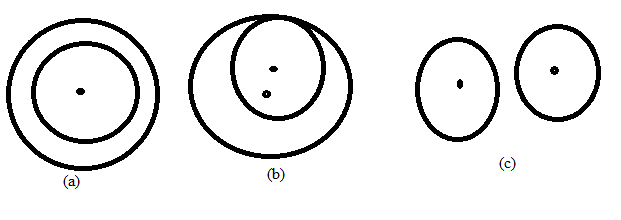Chapter 6.3, Problem 25E### Elementary Geometry for College St...

6th Edition
Daniel C. Alexander + 1 other
ISBN: 9781285195698

#### Solutions

Chapter
Section### Elementary Geometry for College St...

6th Edition
Daniel C. Alexander + 1 other
ISBN: 9781285195698
Textbook Problem
1 views

# For the two circles in figures (a), (b), and (c), find the total number of common tangents (internal and external).To determine

(a)

To find:

To find the total number of common tangents (internal and external).

Explanation

Consider the following figure,

To determine

(b)

To find:

To find the total number of common tangents (internal and external).

To determine

(c)

To find:

To find the total number of common tangents (internal and external).

### Still sussing out bartleby?

Check out a sample textbook solution.

See a sample solution

#### The Solution to Your Study Problems

Bartleby provides explanations to thousands of textbook problems written by our experts, many with advanced degrees!

Get Started

#### Find more solutions based on key concepts